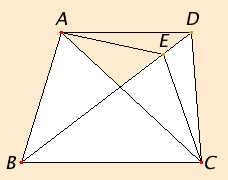# Proposition 39

Equal triangles which are on the same base and on the same side are also in the same parallels.
I.Post.1

Let ABC and DBC be equal triangles which are on the same base BC and on the same side of it. Join AD.I say that AD is parallel to BC.

If not, draw AE through the point A parallel to the straight line BC, and join EC.

I.37

Therefore the triangle ABC equals the triangle EBC, for it is on the same base BC with it and in the same parallels.

C.N.1

But ABC equals DBC, therefore DBC also equals EBC, the greater equals the less, which is impossible.

Therefore AE is not parallel to BC.

Similarly we can prove that neither is any other straight line except AD, therefore AD is parallel to BC.

Therefore equal triangles which are on the same base and on the same side are also in the same parallels.

Q.E.D.

## Guide

This is a partial converse to proposition I.37, only partial since the two triangles ABC and DBC have to be on the same side of the line BC. If they weren’t, then of course AD would not be parallel to BC but instead cross it at the midpoint

#### Use of Proposition 39

This proposition is used in VI.2.